## The Shape of Bits to Come

### by James Miller, G3RUH

ABSTRACT

This tutorial article is about bits, about bandwidth and about control of both.  Lately a number of expressions have crept into amateur radio data transmission, creating both interest and confusion.  What, for example, is the "raised cosine modulation" used on microsats AO-16/18/19?  And what is the RSM-8 on Rudak/AO-21?  What PSK modulation does Fuji-20 use?  Come to that, what is PSK anyway?  This piece is not just "about satellites", or "about packet".  The principles discussed here form the foundation of all data transmission, and ought to be as familiar as Ohms law.  Read on!

Let's come clean at the outset; "raised cosine", RSM-8, PSK and DPSK are the same thing.  They're all forms of PSK - "phase shift keying".

In the amateur environment we are usually trying to send data as fast as possible within a limited AF or RF bandwidth.  This limit may be set by the receiver alone, or it may be due to some statutory, aesthetic or technical requirement.  So a very important part of data transmission concerns the data's spectrum.

Understanding PSK

Let's begin with a statement and explain later:

PSK spectral considerations at RF can be analysed by examining the characteristics of an isolated bit at "DC" or baseband.

I have to start somewhere, so I'll make the assumption that you have in your head an image that "data" is the sort of regular signal you have running down a piece of cable.  On a 'scope it looks like random highs and lows spaced at regular intervals.  Each of these elements is called a "bit", and they flow at the "bit rate", described as 9600 bits/sec or sometimes "9600 baud" depending on the context.  See the waveform "Data In" of Figure 1.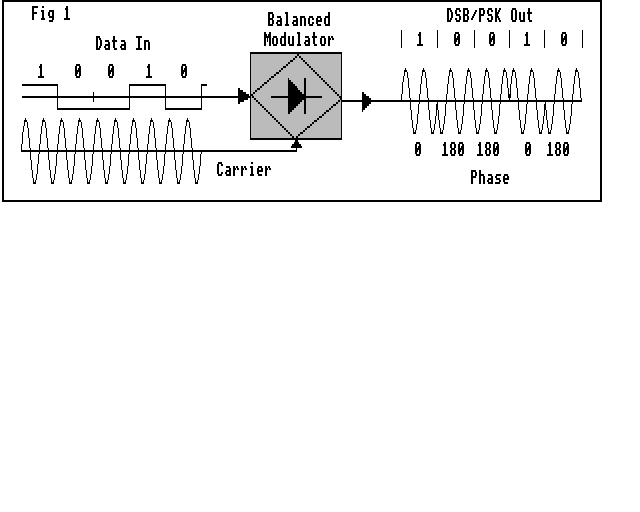Figure 1.  PSK is usually generated by a DSB balanced modulator.  So DC spectrum is simply shif ted to RF.  Data "1"s give carrier phase 0, data "0"s give phase 180.

There are many ways to modulate this data onto an RF carrier, for example ON/OFF keying, carrier FSK, two-tone AFSK and so on.  Here I am concerned with double sideband modulation, DSB.  That is, the data signal drives a balanced modulator whose other input is the RF carrier.  The output then is simply a frequency shifted replica of the input data, much the same as when the input is speech.

Now binary data has only TWO states.  It is either a "1", represented by +1 Volt say, or a "0", represented by -1 Volt.  And that means that the modulated RF carrier also takes only TWO states; either it has phase 0 degrees or 180 degrees.  This is because compared with the +1 Volt level, the -1 Volt inverts the carrier.  That means a 180 degrees phase shift.  Voila!  Binary Phase-Shift-Keying or BPSK.  The term PSK is often used by default, but is actually imprecise as it embraces other shifts as well as 0/180 degrees.  "Antipodal PSK" is correct but long-winded.

It follows then that if we know the spectrum of the "baseband" data signal, then the RF spectrum is just its replica above and below the carrier frequency, because it's DSB, double sideband suppressed carrier.

If in addition, the data signal has amplitude variations, then these will translate into identical amplitude variations at RF.

This is a very important equivalence, and it's worth restating:

"Binary data DSB modulating an RF carrier" and "binary phase shift keying (BPSK)" are exactly the same thing.  If we want to control or analyse the RF spectrum characteristics, we only need to control or analyse the source data characteristics.

Understanding Data Streams and Isolated Bits

We now make a reasonable assumption: the data stream consists of random bits.  If the data is random, for the purposes of analysis we don't then need to know anything about the content of the message stream.  All we need to know is the properties of ONE ISOLATED BIT.  Whatever properties we can discover about the isolated bit will also apply to the average summation of the random assortment of them that make up the stream.

So our problem of spectral considerations at RF can be analysed via the relatively simpler job of examining the characteristics of an isolated bit at source level, DC or, as it is appropriately called, "baseband".

As a communications theory aside, it is worth noting that with efficient communications, that data MUST in fact be random.  For if it were not, that would imply something systematic about it.  So the data could actually be represented or coded more efficiently, resulting in less transmitted bits, which would then have a random distribution.

Understanding Bit, Eyc, & RF Spectrum Plots

Now let's examine some representative cases.  What happens if we modulate the carrier directly with unprocessed rectangular bits?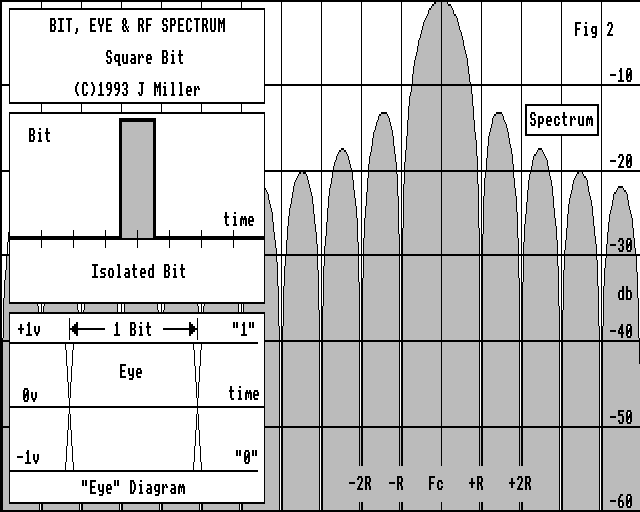Figure 2.  Rectangular bit, its eye and spectrum. See text for details.

Figure 2 shows three things A) an isolated rectangular bit, B) its RF spectrum and C) what the data stream looks like on an oscilloscope.  Of course the bit edges are in reality almost invisibly fast, so I have emphasised them slightly.

A)  Isolated Bit.  The horizontal axis is time, and is marked off in bit intervals.  (Eight are shown).  The vertical is voltage.

B)  RF Spectrum.  The horizontal axis is frequency, and is marked off in units of the bit rate R bps.  For example, if the data rate is 1200 bit/sec (R = 1200), the vertical markers are at 1200 Hz spacing.  The mid point of this spectrum is of course the RF carrier frequency.  The vertical is marked off at 10 dB intervals.  Before modulation, at "DC" or baseband, the audio spectrum is just one half of this, the right of Fc, which therefore represents 0 Hz.

C)  Oscilloscope diagram.

1. The horizontal axis is time, and spans a total of two bits; so it's expanded x4 compared with the isolated bit.  The vertical is voltage.  You are to imagine the 'scope is triggered by a local bit-rate clock.  The resultant pile-up of bits is what you see displayed.  It is the sum of many positive and negative isolated bits, each displaced in time by one bit.
2. If this display is the received waveform, it's what is sampled by the data detector to decide if a "1" or a "0" has been received.  Usually this decision is taken at the mid-time point of the received bit by a sampler.  Above the horizontal line is the regime of "1"s, below "0"s.
3. This display is also universally known as an "eye diagram".  It is a concise representation of the quality of the received signal.  The less confused the trace, the greater the distinction between high and low at the mid-bit sample point, then the more reliable (less error prone) bit detection will be.
4. These traces show no channel noise.  That is to say, the signal to noise ratio is assumed high.  If there were noise, the traces would be blurred.  If the noise were momentarily large enough to cause the waveform accidentally to cross the middle horizontal line, an erroneous detection decision would be made, leading to a received bit error.

Rectangular Bit

Returning to our rectangular bit shape.  Two things are clear from Figure 2; first, the extremely high fidelity of the data waveform and second, the price for this, profligate use of bandwidth.  The diagram shows significant energy spreading well beyond 10 times the data rate.  Indeed, as far away as 30 R (R = bit rate), the sidelobes are still only -40 dB down.  As sufferers from computer hash will know, rectangular bits are effective noise generators.

In some applications (for example spread spectrum communications) this might be the desired result.  In the amateur radio environment where we want to get as much data through the limited bandwidth of our receivers, more finesse is required.

Obviously we need to filter the data stream before transmission, because this will attenuate those sidelobes and constrain the bandwidth.

So the key issue, indeed perhaps the central problem of all data transmission, is "what form should this filter take"?

Performance of a Simple R-C Network Data Filter

We tentatively try a simple filter.  Let's pass the data stream through an R-C network with a -3 dB point equal to half the data rate.  The result is shown in Figure 3.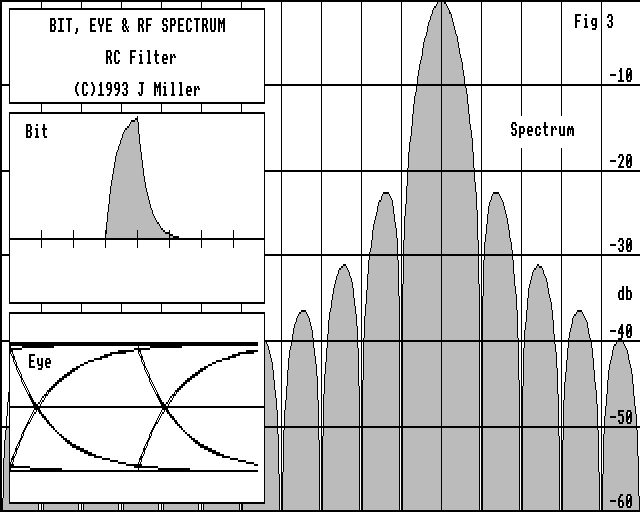Figure 3.  Rectangular bit through R-C filter has classic exponential rise and fall, and RF sidebands are reduced slightly.

Look at the spectrum; while the main lobe is hardly changed, the sidelobes are reduced by 10 dB or more, which is what we wanted.  Look at the isolated bit; it has become rounded.  But note particularly that its duration now exceeds one bit.  In fact it's stretched to roughly two bits long.

This means that successive bits will overlap.  In turn this brings a new potential design problem; inter-symbol interference, or ISI.  Now we find that not only do we want spectrum control, but we have to do it with the constraint that successive overlapping bits must somehow not interfere with each other.

Finally, look at the 'scope trace.  The line at the top corresponds to the sequence 111..., that at the bottom 000.. .  The sweeps from top to bottom are caused by ..10.. and ..01.. transitions and so on.  You can also see that the traces are not confused; highs and lows of successive bits are separable, so there is no significant inter-bit interference.

Inter-Symbol Interference Revealed

In an attempt to attenuate the sidelobes more, let's now double the RC filter's time constant.  That is, the -3 dB frequency is now 1/4 the bit rate (R/4).  See Figure 4.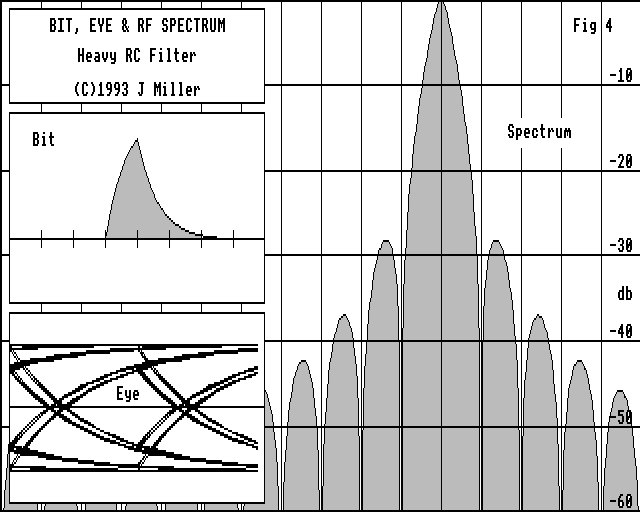Figure 4.  But too much ill-designed filtering gives inter symbol interference (ISI).

Indeed the sidelobes are reduced; but the real casualty can be seen in the 'scope trace.  Under certain conditions (for example the sequence ..010.. or ...101... ) the voltage barely gets half way up to the top line.  Sure, bits are still distinct and detectable, but the noise margin is drastically reduced by some 50%.

Analytically, the reason for this is seen in the isolated bit diagram of Figure 4.  The bit starts off at T = 0.  At T = 1 it reaches its peak.  At T = 2, it hasn't fallen back to zero again, but has a value approximately 1/3rd peak.  This aberration is the sole cause of the inter-symbol interference apparent in the 'scope trace.  At T = 3, 4 etc, the voltage is back to zero again.

From this observation we can formulate the requirement for a bit shape that guarantees no ISI.  There should be a peak of unity at T = 0.  Then at all other exact bit points (T = -2, -1, +1, +2, etc) the isolated bit waveform should be exactly zero.  What happens in between doesn't matter, at least, not from the ISI point of view.

FO-20's Data Filter

An example of a bit shape that meets this requirement is that transmitted by the satellite Fuji-Oscar 20.  The data filter is (I believe) a 3rd order Bessel type with a -3 dB point at 0.532 times the bit rate (0.532 R).  "Bessel" filters driven by square waves have a nice steady rise, and negligible overshoot or ringing.  In electrical circuit terms, the filter is merely 3 resistors, 3 capacitors and an op-amp.  The filter is shown in Figure 5, responses in Figure 6.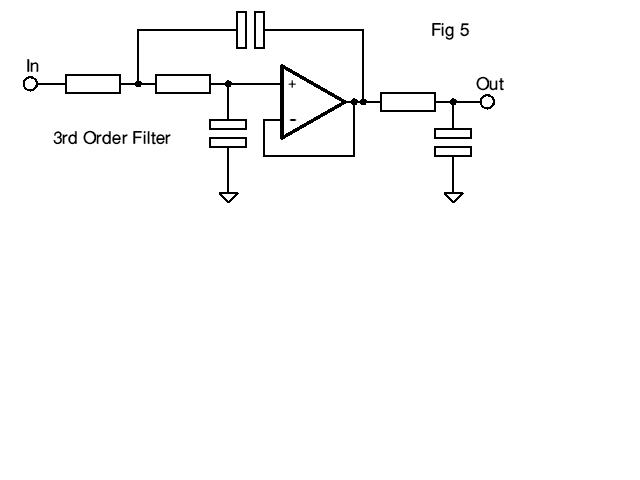Figure 5.  Packet satellite FO-20's 3rd order data filter is similar to this.  Waveshapes as Figure 6.Figure 5.  Characteristics of FO-20 data.  The far-out RF sidelobes are much more rapidly attenuated than in Figure 4, yet there is no confusion between adjacent bits (no ISI).

The spectrum is quite reasonably controlled; 99.9% of the energy is contained within a bandwidth of 1.75 R (as compared with 15 R of the rectangular pulse).  Since the FO-20 bit rate is 1200 bps, this means that most of the signal occupies an effective RF bandwidth of the order of 2.1 kHz, so it stands a fair chance of passing through the SSB receiver filters without significant distortion.

The isolated bit shape is zero at all T points except at one, and this leads to a nice 'scope trace.  The origin of the term "eye diagram" can be seen here, as the trace is supposed to resemble a human eye.  Notice that as a consequence of negligible inter-symbol overlap in the isolated bit, the 'scope trace has perfect bit convergence at the sample point.

The isolated bit has a duration of just 2 bits.  This means that there can be only 22 = 4 trajectories on the 'scope trace (00, 01, 10, and 11).  The 01s and 10s show up as a very clean zero crossover.  If the RF bandwidth were narrower then the isolated bit would span more than 2 bits, so the 'scope trace zero crossings would show dispersion.

And Now - "Raised Cosine"

The term "raised cosine" means several things, a fact which causes a lot of confusion.  A raised cosine is just that.  A cosine function raised above its mid point.  The formula for a raised cosine is: (1 + cos x) / 2.  Drawn as a graph, it's merely a shape.  But it has a number of analytical properties that make it convenient to employ.  These are simplicity left-right and upper-lower symmetries.  (See Figure 7a).Figure 7a.  A raised cosine shape.  There's nothing mysterious about a raised cosine.  Just get out your calculator and plot: y = (1 + cos x) / 2 for x = -180 to 180 degrees!

A Raised Cosine Bit

"Raised cosine" can for example, describe the shape of the isolated bit - see Figure 7.  Since the isolated bit is cosine shaped, the 'scope trace is composed entirely of sine waves and straight lines.  Indeed, the bit sequence ..10101010.. actually creates a pure tone at a frequency R/2 at baseband, or at RF, two frequencies spaced by +/- R/2.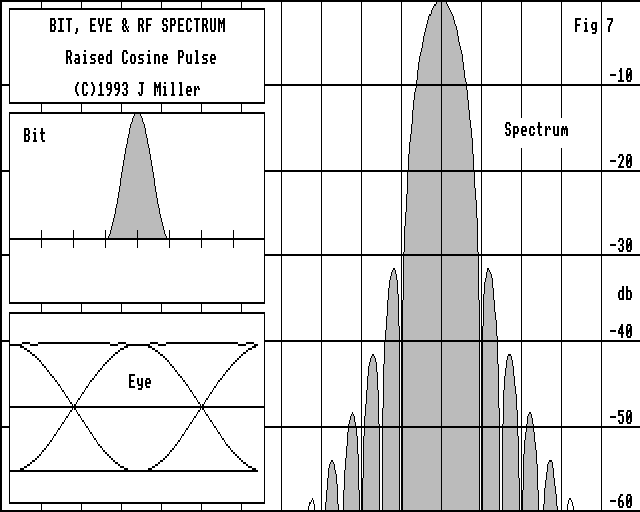Figure 7.  Raised cosine bit TIME shape used (in principle) on PacSats AO-16/ WO-18/ LO-19.

The isolated bit shape is very similar to FO-20's, occupying 2 bits.  Not surprisingly the spectrum is very similar too.  In fact 99.9% of the energy is contained within 1.69 R (compared with 1.75 R for FO-20).  An important practical point to remember is that the shape of the bit is completely specified in time.  This means that it can be precisely generated using real finite hardware, such as a look-up table.  The microsats AO-16/WO-18/LO-19 use a filter not unlike this.

The Raised Cosine Spectrum

Alternatively "raised cosine" can also be used to describe the shape of the spectrum.  This is illustrated in Figure 8.  It doesn't look much like a raised cosine spectrum because of the logarithmic scale, but the response is unity (0 dB) at f = 0, 0.5 (-6 dB) at f = R/2 and exactly zero at f = R, the bit rate.  99.9% of the energy is contained within 1.56 R.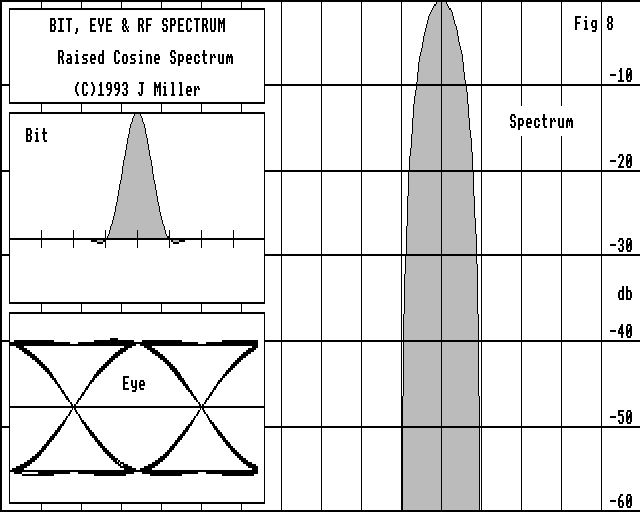Figure 8.  Raised cosine SPECTRUM shape.  There is a family of these shapes, with the raised cosine part centred on the middle of each side of the spectrum.  This synthesis gives a range of shapes from the gentle one shown above to the theoretical minimum "brick wall" (Bandwidth = data rate).

Because the spectrum is absolutely band limited to +/- R, the isolated bit shape that gives rise to that spectrum has infinite time duration.  This means that it cannot be synthesised with real finite hardware.  On the other hand, as Figure 8 shows, it has negligible amplitude beyond T = +/- 2 bits, so that discarding the tails has little effect other than to bring in some very minor spectral sidelobes.  So synthesis turns out to be highly practical.

You will also notice that the isolated bit also has precisely the desired properties for zero inter-symbol interference.  Is this fortuitous?  Certainly not!  In fact it is a direct consequence of the two symmetries of the cosine shape mentioned earlier.  That's why the raised cosine spectrum is so widely used in data transmission system design.

9600 Baud Packet Radio & UOSAT/OSCARs 22/23 - A Narrowband Raised Cosine Spectrum

As a practical example of "raised cosine" spectrum shaping consider Figure 9.  Here only the middle 3/8ths of each half of the spectrum is given a raised cosine shape.  From f = 0 to 5/16 R the spectrum is flat (0 dB), from 5/16 R to 11/16 R it follows the cosine shape, and from 11/16 R onwards the spectrum is zero.Figure 8.  UOSATs 14, 22 and KitSat Oscar-23 have almost "rectangular" raised cosine spectra (see text).  The narrow single main spectrum lobe implies a bit that is some 8 bit periods long.  There is no ISI

This is used in the baseband filtering of UoSat/KitSat/OSCARs 22/23 which use the G3RUH 9600 baud Packet Radio modem [ref 1].  The isolated bit shape spans some eight bit periods.  That is to say a bit transmitted "now" will actually have an influence on up to eight other bits, four before and four after "now".  Hence the title of this article!  Note also that the isolated bit has the desired form for minimising inter-symbol interference.  A peak at T = 0, zero at all other T points.  So the received "eye" shows good convergence at the sample point.

The spectrum is almost rectangular ("brick-wall") and sidelobe free.  99.9% of the spectral energy is contained within 1.2 R, or +/- 5.6 kHz at 9600 bps.

The zero-crossing dispersion is a direct consequence of the narrow bandwidth, and great care is needed in the design of the receiver bit-clock recovery circuits that perform time bit detection.  You can see that sampling only 1/8th bit away from the convergence point means bit detection half way down to the zero threshold, and so the instantaneous noise tolerance would be reduced by 6 dB.  Narrow bandwidth bit-clock recovery circuits are essential.  For implementation details see [ref 1].

The actual signals transmitted by UoSat/KitSat are slightly different to that shown; they are pre-adjusted (in time) so that when they pass through a real bandwidth limited receiver the final "eye" is as illustrated.

In the G3RUH/PacComm/Kantronics/Symek/Tasco Telereader embodiment of this modem, transmit bit-shape is looked up from a table of values in an EPROM and passed to a DAC.  This data filter is known as an FIR for Finite Impulse Response.  UO-22/23 use a tapped digital delay line (shift register) and summing op-amp; EPROMs do not always travel well in spacecraft.  This mechanisation of an FIR is called a Transversal Filter.

In UoSat/KitSat the signal also FM modulates the carrier (as opposed to PSK).  FM is simple to generate, much simpler to process on receive in the presence of mistuning such as Doppler shift or receiver drift, and is very robust.  Though FM does require slightly stronger signals, 6-8 dB perhaps, this is not a disadvantagous for amateurs where we are rarely power limited.

Finally note that this precision data shape could trivially generate BPSK via a DSB balanced modulator.  Such PSK would have all the narrow-band and non-ISI attributes detailed above.

Transmitter and Receiver Filtering in Series

So far discussion has mostly been about spectra and bit shapes in association with transmitters.  It was implicit that the receiver was infinitely wideband.  For analytic purposes, if there were any RX filtering, we have assumed it to be merged with the transmit filtering.

In many amateur applications this is indeed the case.  For example, the current flock of PSK packet satellites (Oscar-16 etc) confine their bit rates to that which can be passed through a typical SSB receiver.  RTTY users assume the same thing.

We now ask the important question; if the overall filtering were to be deliberately split between transmitter and receiver, what should the form of the filters be?

We know from all the forgoing what kind of overall response is required.  But how should we partition it?

Obviously, the overall response can be apportioned in any ratio whatsoever.  However one can prove that in the presence of random noise the optimum split, so as to maximise detector signal-to-noise ratio, is that with exactly half the frequency response in the transmitter, and the other half in the receiver.  These are known as a "matched filter pair".

Conceptually, one can first imagine a desired overall frequency response dictated by inter-symbol interference requirements, spectral constraints and implementation ease.  Then as it were, you take the "square root" of this, and put the resulting filter into both transmitter and receiver.

AO-21/RUDAK-2 "RSM" Bit Shaping

A very good example of this is the bit shaping scheme used on one of the RUDAK-2 links of AO-21 [ref 2].  Winningly dubbed "RSM" Rectangular Spectrum Modulation by its creator, its transmit filter properties are shown in Figure 11 (left).  Both transmitter and receiver have essentially identical filters.  This bit shape is used to phase modulate (BPSK) the RF carrier.  Bit rate is R = 9600 bps.

Figure 11a & b.  Performance of RUDAK-2's "RSM" TX filter (a) and signal after passing through complementary "matched" TX + RX filters (b).  The eye is as wide as possible at the detection point, and regardless of neighbours, all bits have the same amplitude at this instant.  (i.e. no ISI)

99.9% of the spectral energy is contained within a bandwidth of 1.5 R, which is consistent with the isolated bit span of about 3 bits.  This means that there are about 23 = 8 trajectories in the 'scope trace, which can be seen quite clearly.  It is notable that this isolated bit shape is created using analogue filtering rather than more accurate and repeatable digital synthesis.

The transmit filter (Figure 10) has 3 sections, comprising a) a Cauer Chebyscheff type CC0315/41 which is flat up to a frequency f = R/2, and then plunges down to the zero at f = R.  But above this there is a rise to only -15 dB.  So part b) is a 3rd order Butterworth that's smooth to the -3 dB point at f = R/2, and then -18 dB at f= R and -36 dB at f = 2R; c) an "all-pass" network that has a dead flat frequency response, but a tapered phase characteristic which is used to flatten out the filter delay vs. frequency curve, called "linear phase".  Linear phase filters result in a transmit bit shape that is left-right symmetric.  This filter is not driven directly by the rectangular bit stream, but by short + or - pulses of polarity corresponding to the data bits' polarity.Figure 10.  Data filter used in RUDAK-2 "RSM" transmitter.  This comprises three cascaded sections; a 3rd order Cauer, a 3rd order Butterworth and an all-pass (refer to text).  This transmit filter is used in association with an almost identical filter at the receiver.  These filters are thus "matched".  For spectrum and bit shapes see Figure 11.

When cascaded with an identical analogue filter in the receiver, the overall response is as per Figure 11b.  The overall isolated bit shape is almost perfect, spanning some 5 bits with remarkable symmetry.  The 'scope trace shows an excellent eye.  The sample point convergence has about +/- 8% scatter due to the slight non-zero values of the isolated bit shape at T = -2 and T = +2.

The Future?

The 9600 baud RSM-PSK implementation is near optimal in performance, probably within 1 dB of the theoretical limit.  [approx. 20 Log (1 - 8/100)]

It is flying on RUDAK-2/AO-21 so AMSAT experimenters (i.e. YOU) can evaluate the effectiveness of high speed PSK with or without coding [ref 3] from the associated on-board Harris RTX2000 RISC processor.  This will help determine what is practical and realistic for amateur spacecraft and other digital links of the future.

The G3RUH 9600 baud FM system is not only in daily use by many satellite stations on UO-22/KO-23, but also hundreds of terrestrial packet radio links world wide.  Indeed, the outstanding German packet radio [ref 4] network thrives on it.  The modem represents a design that pushes the fastest possible binary data through a basic FM radio.  9600 bps throughput is astonishing to behold, especially on a full duplex satellite link.

Who will predict that one day even our voice repeater links will be entirely digital.  I will!

References

1.  Miller J R, "9600 baud Packet Radio Modem Design", Proc. 7th ARRL Computer Networking Conference, 1988 Oct, pps 135-140

2.  Meinzer K & Haas W, "RUDAK 2 - The Radio Links", Amsat-DL Journal No. 1/17, March 1990 pps 9-12. (German). English translation in Oscar News No. 83, 1990 June, pps 16-21

3.  Miller J R, "Shannon, Coding and the Radio Amateur", Oscar News No. 81, 1990 Feb, pps 11-15

4.  Rech W & Kneip J, "The German (Central European) Packet Radio Network: An Overview", Proc. 11th ARRL Computer Networking Conference, 1992 Nov, pps 52-56.

```This placement:  ftp.amsat.org  /amsat/articles/g3ruh/a108.zip
Date:            1995 Dec 19

First placement: Amsat-UK's Oscar News,  1991 Apr   No.88 p.29-37
Corrections:     Minor edits
Size:            26,000 bytes  4200 words  560 lines
```